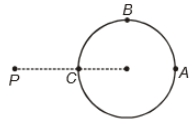A hollow conducting sphere is placed in an electric field produced by a point charge placed at P as shown. Let ${V}_{A},{V}_{B},{V}_{C}$ be the potentials at points A,B and C respectively. Then1. ${V}_{A}<{V}_{B}<{V}_{C}$

2. ${V}_{A}>{V}_{B}>{V}_{C}$

3. ${V}_{C}>{V}_{B}={V}_{A}$

4. ${V}_{A}={V}_{B}={V}_{C}$

Subtopic:  Electric Potential |
To view explanation, please take trial in the course below.
NEET 2022 - Target Batch - Aryan Raj Singh
To view explanation, please take trial in the course below.
NEET 2022 - Target Batch - Aryan Raj Singh

Difficulty Level:

Four particles each having charge q are placed at the vertices of a square of side a. The value of the electric potential at the midpoint of one of the side will be

1.  0

2.  $\frac{1}{4\pi {ϵ}_{0}}\frac{2q}{a}\left(2+\frac{2}{\sqrt{5}}\right)$

3.  $\frac{1}{4\pi {ϵ}_{0}}\frac{2q}{a}\left(2-\frac{2}{\sqrt{5}}\right)$

4.  $\frac{1}{4\pi {ϵ}_{0}}\frac{2q}{a}\left(1+\frac{1}{\sqrt{5}}\right)$

Subtopic:  Electric Potential |
To view explanation, please take trial in the course below.
NEET 2022 - Target Batch - Aryan Raj Singh
To view explanation, please take trial in the course below.
NEET 2022 - Target Batch - Aryan Raj Singh

Difficulty Level:

If E be the electric field inside a parallel plate capacitor due to Q and -Q charges on the two plates, then electrostatic force on plate having charge -Q due to the plate having charge +Q will be

(1) -QE

(2) $\frac{-QE}{2}$

(3) QE

(4) $\frac{-QE}{4}$

Subtopic:  Combination of Capacitors |
To view explanation, please take trial in the course below.
NEET 2022 - Target Batch - Aryan Raj Singh
To view explanation, please take trial in the course below.
NEET 2022 - Target Batch - Aryan Raj Singh

Difficulty Level:

If W be the amount of heat produced in the process of charging an uncharged capacitor then the amount of energy stored in it is

(1) 2W

(2) $\frac{W}{2}$

(3) W

(4) zero

Subtopic:  Energy stored in Capacitor |
To view explanation, please take trial in the course below.
NEET 2022 - Target Batch - Aryan Raj Singh
To view explanation, please take trial in the course below.
NEET 2022 - Target Batch - Aryan Raj Singh

Difficulty Level:

A metallic sphere of capacitance ${C}_{1}$, charged to electric potential ${V}_{1}$ is connected by a metal wire to another metallic sphere of capacitance ${C}_{2}$ charged to electric potential ${V}_{2}$. The amount of heat produced in the connecting wire during the process is

(1)$\frac{{C}_{1}{C}_{2}}{2\left({C}_{1}+{C}_{2}\right)}{\left({V}_{1}+{V}_{2}\right)}^{2}$

(2) $\frac{{C}_{1}{C}_{2}}{2\left({C}_{1}+{C}_{2}\right)}{\left({V}_{1}-{V}_{2}\right)}^{2}$

(3) $\frac{{C}_{1}{C}_{2}}{{C}_{1}+{C}_{2}}{\left({V}_{1}-{V}_{2}\right)}^{2}$

(4) zero

Subtopic:  Energy stored in Capacitor |
To view explanation, please take trial in the course below.
NEET 2022 - Target Batch - Aryan Raj Singh
To view explanation, please take trial in the course below.
NEET 2022 - Target Batch - Aryan Raj Singh

Difficulty Level:

The electric potential at the surface of a charged solid sphere of insulator is 20V. The value of electric potential at its centre will be

1.  30V

2.  20V

3.  40V

4.  Zero

Subtopic:  Electric Potential |
To view explanation, please take trial in the course below.
NEET 2022 - Target Batch - Aryan Raj Singh

Difficulty Level:

The capacitance of a parallel plate capacitor is C. If a dielectric slab of thickness equal to one-fourth of the plate separation and dielectric constant K is inserted between the plates, then new capacitance become

1. $\frac{KC}{2\left(K+1\right)}$

2. $\frac{2KC}{K+1}$

3. $\frac{5KC}{4K+1}$

4. $\frac{4KC}{3K+1}$

Subtopic:  Dielectrics |
To view explanation, please take trial in the course below.
NEET 2022 - Target Batch - Aryan Raj Singh
To view explanation, please take trial in the course below.
NEET 2022 - Target Batch - Aryan Raj Singh

Difficulty Level:

The electric potential at a point at distance 'r' from a short dipole is proportional to

(1) ${r}^{2}$

(2) ${\mathrm{r}}^{-1}$

(3) ${r}^{-2}$

(4) ${r}^{1}$

Subtopic:  Electric Potential |
To view explanation, please take trial in the course below.
NEET 2022 - Target Batch - Aryan Raj Singh
To view explanation, please take trial in the course below.
NEET 2022 - Target Batch - Aryan Raj Singh

Difficulty Level:

A hollow charged metal spherical shell has radius R. If the potential difference between its surface and a point at a distance 3R from the center is V, then the value of electric field intensity at a point at distance 4R from the center is

1.  $\frac{3\mathrm{V}}{19\mathrm{R}}$

2.  $\frac{\mathrm{V}}{6\mathrm{R}}$

3.  $\frac{3\mathrm{V}}{32\mathrm{R}}$

4.  $\frac{3\mathrm{V}}{16\mathrm{R}}$

Subtopic:  Electric Potential |
To view explanation, please take trial in the course below.
NEET 2022 - Target Batch - Aryan Raj Singh
To view explanation, please take trial in the course below.
NEET 2022 - Target Batch - Aryan Raj Singh

Difficulty Level:

Capacitors  are connected in series across a source of emf 20KV. The potential difference across ${C}_{1}$ will be

(1) 5 KV

(2) 15 KV

(3) 10 KV

(4) 20 KV

Subtopic:  Combination of Capacitors |
To view explanation, please take trial in the course below.
NEET 2022 - Target Batch - Aryan Raj Singh
To view explanation, please take trial in the course below.
NEET 2022 - Target Batch - Aryan Raj Singh

Difficulty Level: